Representing and manipulating data in computers

Start this free course now. Just create an account and sign in. Enrol and complete the course for a free statement of participation or digital badge if available.

Free course

# 7 Binary arithmetic

Study note: You may like to have the Numeracy Resource (attached below) to hand as you study Section 7. It offers extra practice with the manipulations, and you may find this useful.

View document [Tip: hold Ctrl and click a link to open it in a new tab. (Hide tip)]

Pairs of binary digits are added according to the rules

• 0 + 0 = 0

• 0 + 1 = 1

• 1 + 1 = 10, which is 0 and carry 1.

This last rule arises from the fact that 10 in binary is the equivalent of denary 2.

In order to be able to add pairs of binary integers which may consist of several bits, one further rule is needed to allow for the fact that a carry may have to be added into a pair of bits. This rule is

1 + 1 + 1 = 11

which is 1 and carry 1. (Remember that 11 in binary is equivalent to 3 in denary.)

The following example shows how two 8-bit binary integers are added.

### Example 6

Add the 8-bit binary integers 0101 1100 and 0110 1011.

First the two integers are written in columns under each other: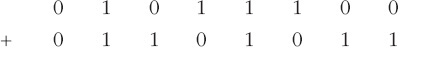Starting with the rightmost (least-significant) bit from both integers and using the above rules gives 0 + 1 = 1, so the rightmost bit of the result is 1. Similarly the next two bits (working from right to left) are also 1. The fourth bit is found from 1+1 and so is 0 with a carry of 1. So far, therefore, the result is: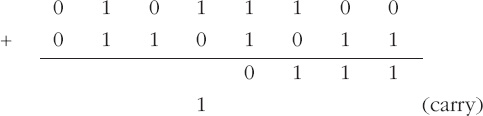The next bit is found from 1 + 0 + 1 and so is 0 with a carry of 1. Similarly, the next bit of the result is 0 with another carry of 1. The result so far is: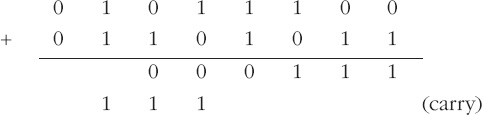The next bit of the result is found from 1 + 1 + 1, which is 1 with a carry of 1. The last (most-significant) bit is therefore 0 + 0 + 1, which is 1. So the final result is: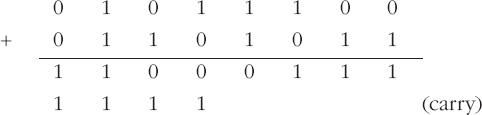Therefore the sum of 0101+1100 and 0110+1011 is 1100+0111.

This can be checked by converting each integer to denary: 0101+1100 is 92, 0110+1011 is 107 and 1100+0111 is 199, which checks.

There is no need to consider the addition of more than two binary integers at once, because in computers integers are generally added two at a time. If three integers are to be added, the first two are added and then the third is added to their sum.

### Activity 23 (Self assessment)

Add the 4-bit binary integers 1011 and 0011. Check your result by converting all integers to denary.

Add the 8-bit binary integers 0110+1100 and 0001+0110.

(a)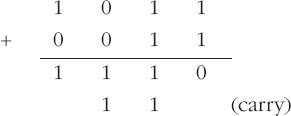So the answer is 1110. Check: 10112 = 1110; 00112 is 310; 11102 is 1410, which checks. (Note that the subscript convention is very useful here in helping to distinguish between binary and denary numbers.)

(b)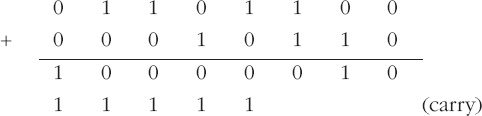Sometimes when two 8-bit integers are added the result cannot be contained in an 8-bit binary word. An example is: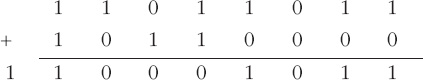where 9 bits are needed to hold the result (because it is greater than denary 255). A similar outcome can occur with binary integers of lengths other than 8 bits. The extra bit generated in the result is called a carry bit. (There can, of course, be intermediate carry bits between two adjacent columns of the sum, but when talking about the addition of two binary integers the term ‘carry bit’ is usually taken to refer to an extra bit generated when the two most-significant bits are added.)

The hardware of most processors has a means of indicating that a carry bit has been generated; it is up to the software to ensure that this carry bit is dealt with appropriately.